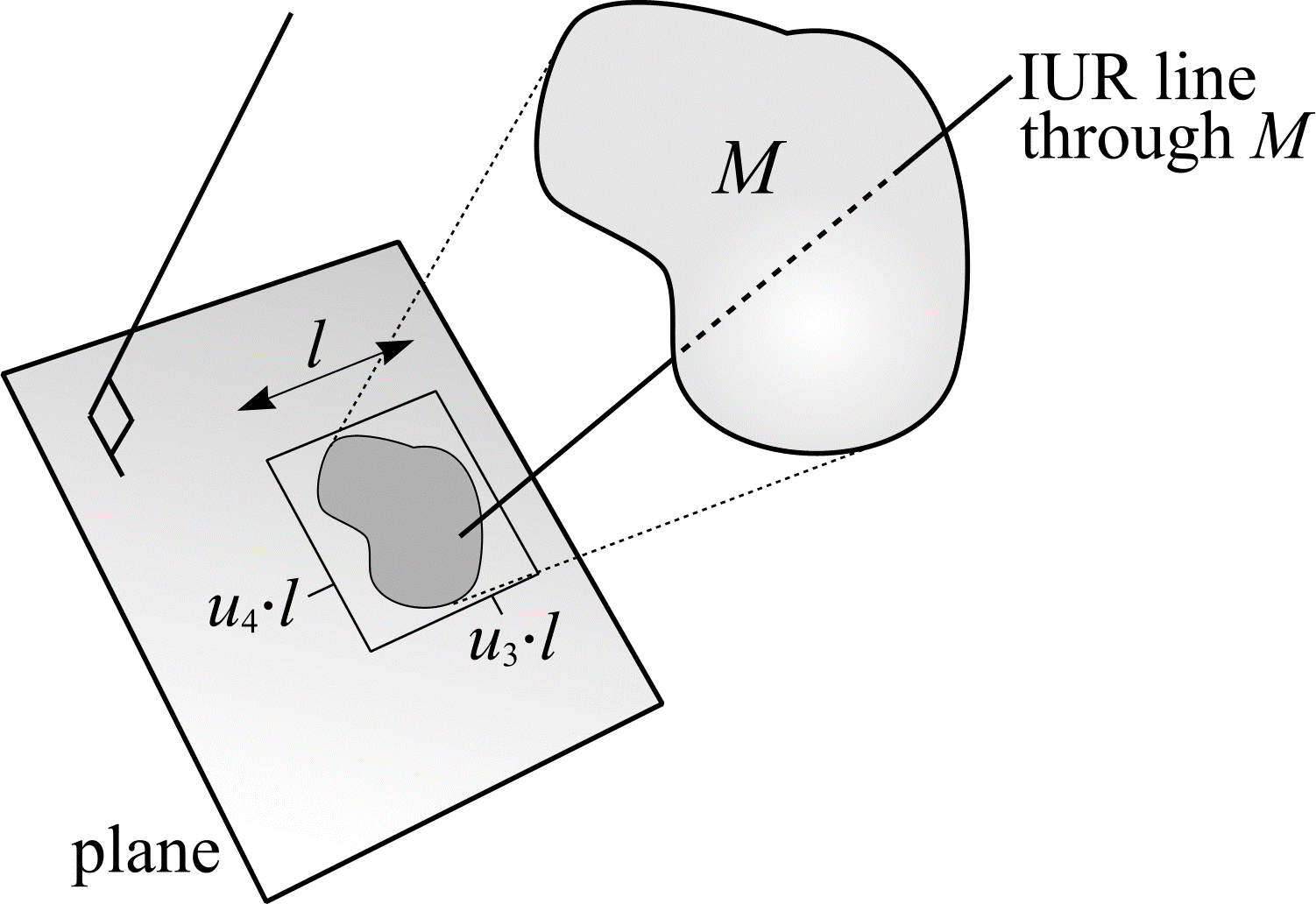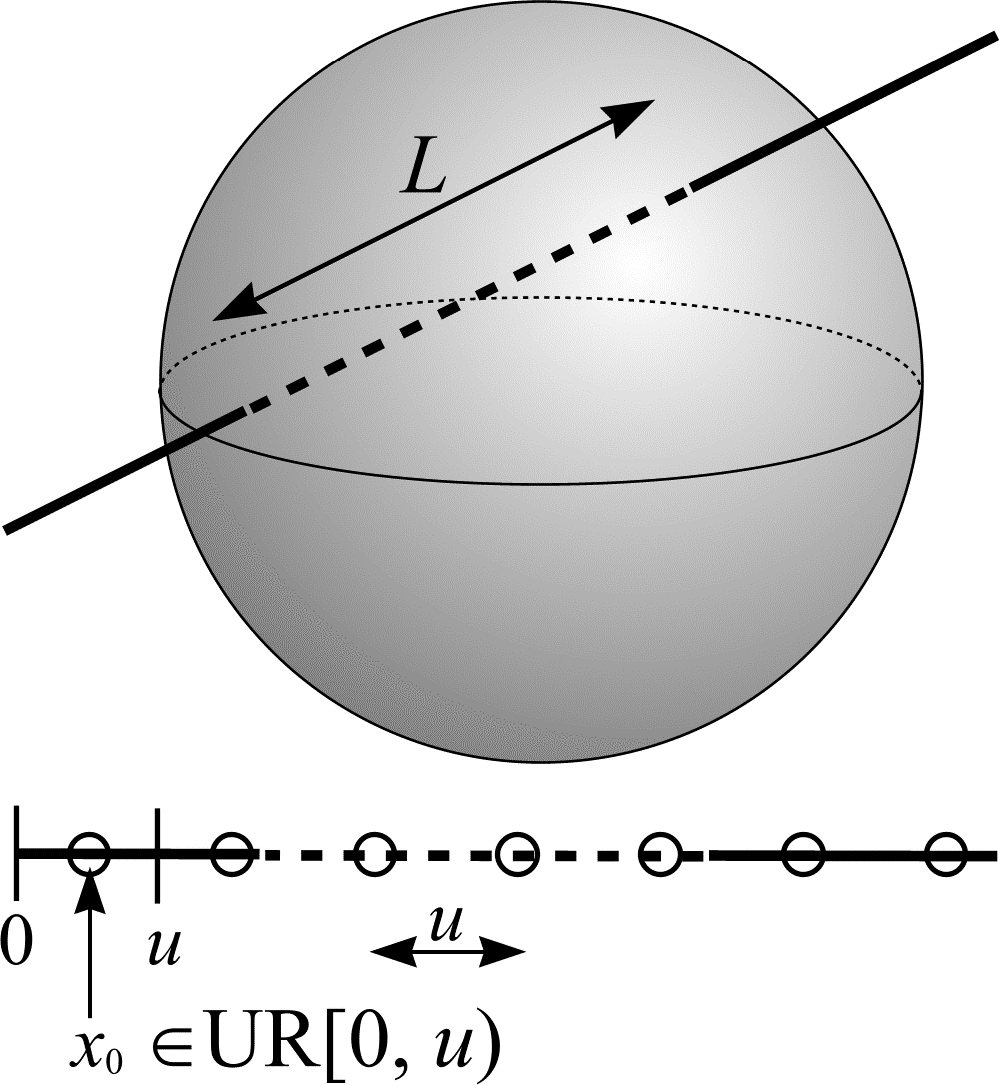# Surface density

Equation (43) says that the length of $$C$$ per unit volume is equal to twice the number of transects between $$C$$ and the test system, per unit area of test system. The dual situation can be conceived if the test system is replaced by a stationary, bounded object in $$\real^{3}$$, $$M$$, whose surface area is $$S$$ and whose volume is $$V$$. Suppose $$S$$ and $$V$$ are unknown. $$L/V$$ is then the length of $$C$$ lying within $$M$$, while $$\mathbf{E}Q/A$$ becomes the number of intersections between $$C$$ and $$M$$, per unit area of $$M$$. By an additional change of notation of $$A$$ into $$S$$ and of $$Q$$ (transect of $$C$$ with a plane) into $$I$$ (intersection produced by $$C$$ in a surface) the following is obtained:

\begin{align} \frac{L}{V} &= 2 \cdot \frac{\mathbf{E}Q}{A} \\ \iff \frac{A}{V} &= 2 \cdot \frac{\mathbf{E}Q}{L} \\ \iff \frac{S}{V} &= 2 \cdot \frac{\mathbf{E}I}{\mathbf{E}L}. \end{align} \tag{45}

Equation (45) determining the surface density, $$S/V$$, of $$M$$ is another fundamental equation of stereology originally obtained by Saltykov (1945). In practise, the (test) curve $$C$$ is replaced by an IUR test system of test lines. $$I$$ and $$L$$ are random variables. $$I$$ denotes the number of intersections between the object surface and the test lines, $$L$$ the length of test lines falling within the object.

An IUR line in $$\real^{3}$$ that passes through $$M$$ can be generated as follows. Let $$u_{1} \in \mathrm{UR}[0, 1)$$, $$u_{2} \in \mathrm{UR}[0, 1)$$, $$u_{3} \in \mathrm{UR}[0, 1)$$ and $$u_{4} \in \mathrm{UR}[0, 1)$$. Construct a line or isotropic axis with orientation $$\theta = \cos^{-1}(1 - 2u_{1})$$ and $$\phi = \pi u_{2}$$. As shown in Figure 16, fix a plane perpendicular to the isotropic axis and project the object, $$M$$, onto that plane. Draw a square on the plane (side length $$l$$), which contains the projection of $$M$$. From a point that has UR position within the square, namely $$(u_{3}\cdot l, u_{4}\cdot l)$$, draw a second axis perpendicular to the plane. If this axis hits $$M$$ (i.e. the point $$(u_{3}\cdot l, u_{4}\cdot l)$$ is contained within the projection of $$M$$), then it can be considered an IUR line in $$\real^{3}$$ that passes through $$M$$. Otherwise the procedure must be restarted with 4 new random numbers.Figure 16: The construction of an IUR line.

Suppose a UR systematic set of points, a distance $$u$$ apart, are positioned along each IUR test line. The length of test line associated with each point, $$l/p$$, is $$u$$. If the expected number of points falling within the object is $$\mathbf{E}P$$, the length of $$L$$ is $$\mathbf{E}P \cdot (l/p)$$ (see Figure 17). Therefore, an estimate of $$S/V$$ can be obtained from a single throw of the IUR test line system:

\begin{align} \text{est } \frac{S}{V} &= 2 \cdot \frac{I}{L} \\ &= 2 \cdot \frac{I}{\left(\frac{l}{p} \cdot P \right)}. \end{align} \tag{46}Figure 17: A method for estimating the length, $$L$$, of test line falling within an object. In this example, the object is a sphere and an unbiased estimate of $$L$$ is 3$$u$$.

## References

SALTYKOV, S. A. Stereometric Metallography, 1st edn. (In Russian). State Publishing House for Metals Sciences, Moscow (1945).